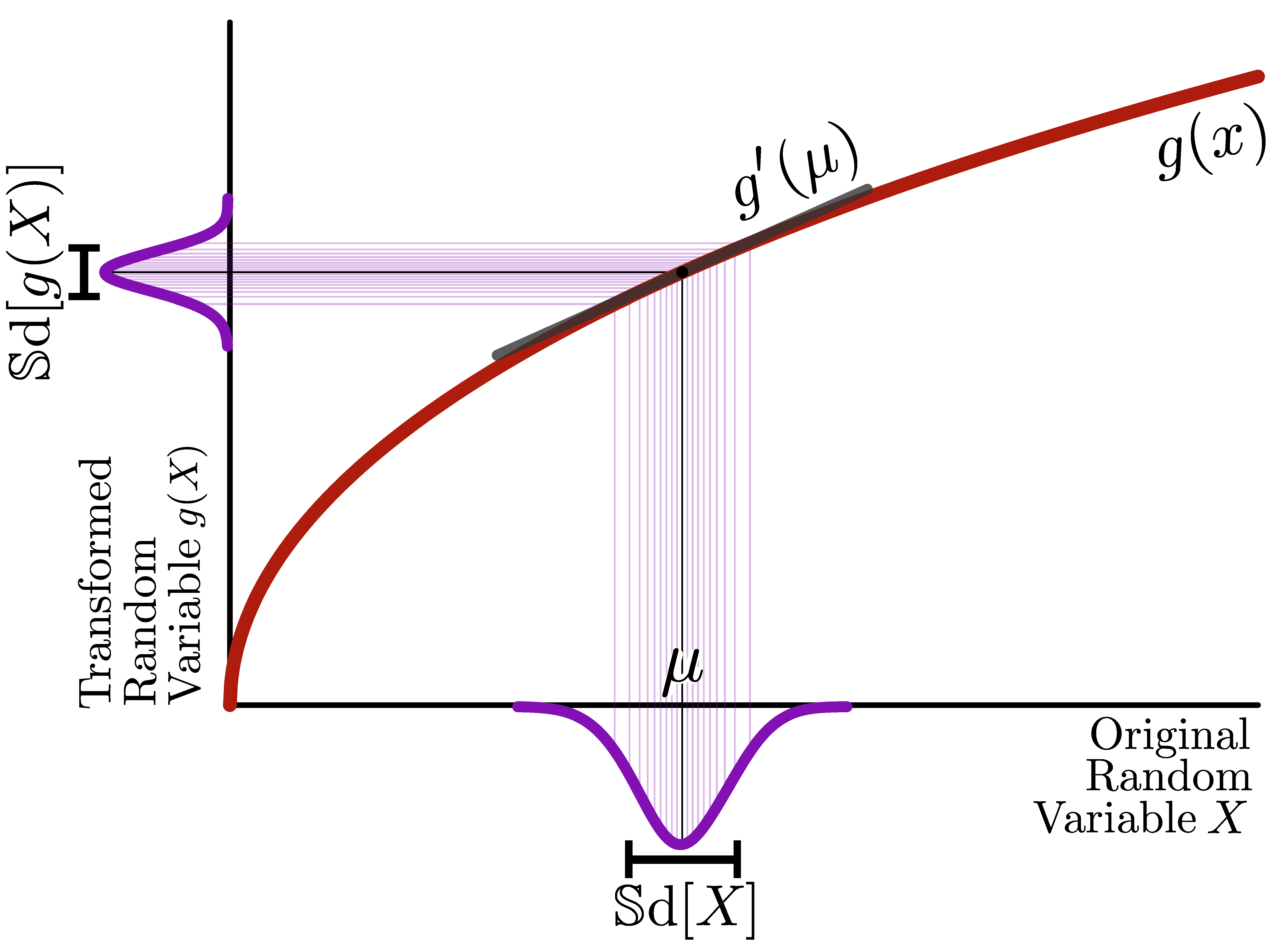# What is the delta method?

Find the standard deviation of $g(X)$

The delta method is a simple trick to find the standard deviation of a transformed random variable.If we apply a differentiable function $$g$$ to a random variable $$X$$ with mean $$\mu$$, the standard deviation of the transformed random variable $$g(X)$$ can be approximated by $\mathbb{S}\text{d}[g(X)] \approx a\; \mathbb{S}\text{d}[X],$ where $$a = |g'(\mu)|$$ is the slope of $$g$$ at $$\mu$$.

### And what about variance stabilizing transformations?

Now consider a set of random variables $$X_1, X_2, \cdots$$ whose variance and means are related through some function $$v$$, i.e. $$\mathbb{V}\text{ar}[X_i] = v(\mu_i)$$, or equivalently $$\mathbb{S}\text{d}[X_i] = \sqrt{v(\mu_i)}$$. Then we can find a variance-stabilizing transformation $$g$$ by requiring constant standard deviation, $$\mathbb{S}\text{d}[g(X_i)] = \text{const.}$$, which using the above approximation becomes $g'(\mu) = \frac{\text{const}}{\sqrt{v(\mu)}},$ and can be solved by integration.

### Example

The Gamma-Poisson distribution (also called Negative binomial) with mean $$\mu$$ and overdispersion $$\alpha$$ implies a quadratic mean-variance relation $\mathbb{V}\text{ar}[X] = v(\mu) = \mu + \alpha \mu^2.$ Our goal is to find a function $$g$$ for which $\mathbb{S}\text{d}[g(X)] \approx \text{const.}$ The delta method approximates the standard deviation of a transformed random variable as $\mathbb{S}\text{d}[g(X)] \approx |g'(\mu)|\;\mathbb{S}\text{d}[X].$ We can require the left side to be constant and solve for $$|g'(\mu)|$$: \begin{equation} \begin{aligned} |g'(\mu)|\;\mathbb{S}\text{d}[X] &= \text{const.} \\ g'(\mu) &= \frac{\text{const.}}{\mathbb{S}\text{d}[X]} = \frac{\text{const.}}{\sqrt{v(\mu)}} \end{aligned} \end{equation}

Given the derivative $$g'$$, we can use integration to identify the functional form of our transformation (note that without loss of generality, we can plug in $$1$$ for the constant, as the value does not affect the variance stabilization property.) \begin{equation} \begin{aligned} g(\mu) &= \int{\frac{1}{\sqrt{v(\mu)}} \text{d}\mu} \\ &= \int{\frac{1}{\sqrt{\mu+\alpha \mu^2}}\text{d}\mu} \\ &= \frac{2}{\sqrt{\alpha}} \operatorname{asinh}\left(\sqrt{\alpha \mu}\right) \\ &= \frac{1}{\sqrt{\alpha}} \operatorname{acosh}\left(2 \alpha \mu +1\right). \end{aligned} \end{equation} You can check the integration using Wolfram Alpha.

If there is no overdispersion ($$\alpha = 0$$), the acosh transformation reduces to the well-known square root variance stabilizing transformation for Poisson random variables $\begin{equation} \lim_{\alpha \to 0} g(\mu) = 2\sqrt{\mu}. \end{equation}$